•matlabmatlab
• 对于偏微分方程来说，尽管在某些意义上是等价的，其数值方法的效果却可以很不相同，正是因为这个原因，用一阶系统极小二乘方法来处理偏微分方程式有意义的。最简单的一阶椭圆系统是柯西利曼方程，本文对这一系统的差...
•自动控制
• 1. 一阶系统 2. 一阶系统输出响应的求法 在零初始条件下，利用拉氏变换或直接求解微分方程，可以求得一阶系统在典型输入信号作用下的输出响应。 3. 一阶系统的单位脉冲响应 3. 时间常数对时间响应的...
1. 一阶系统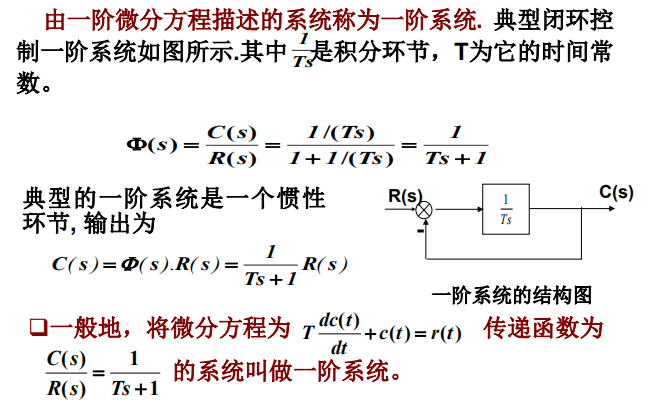2. 一阶系统输出响应的求法
在零初始条件下，利用拉氏变换或直接求解微分方程，可以求得一阶系统在典型输入信号作用下的输出响应。
3. 一阶系统的单位脉冲响应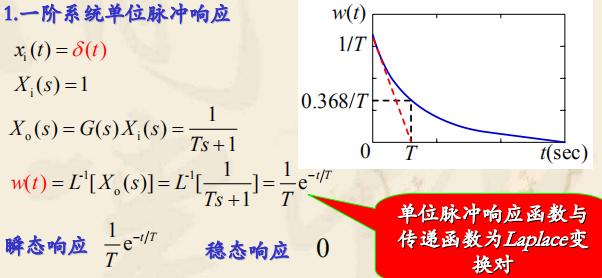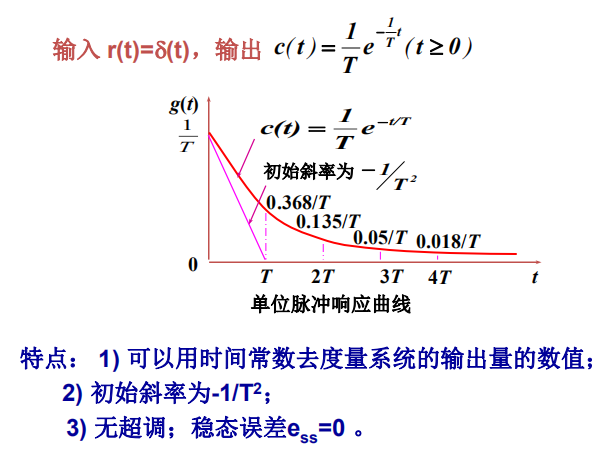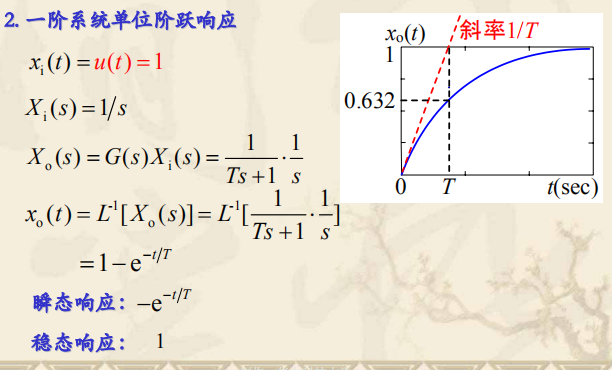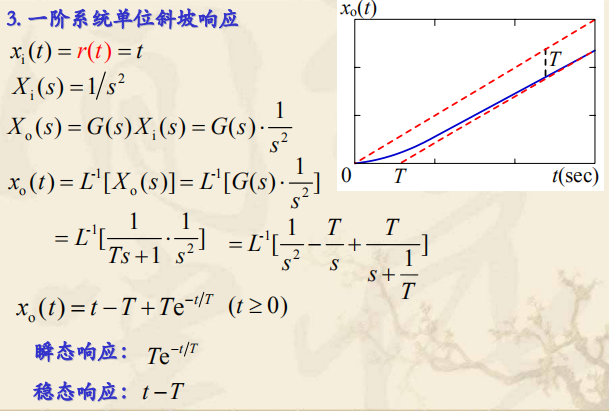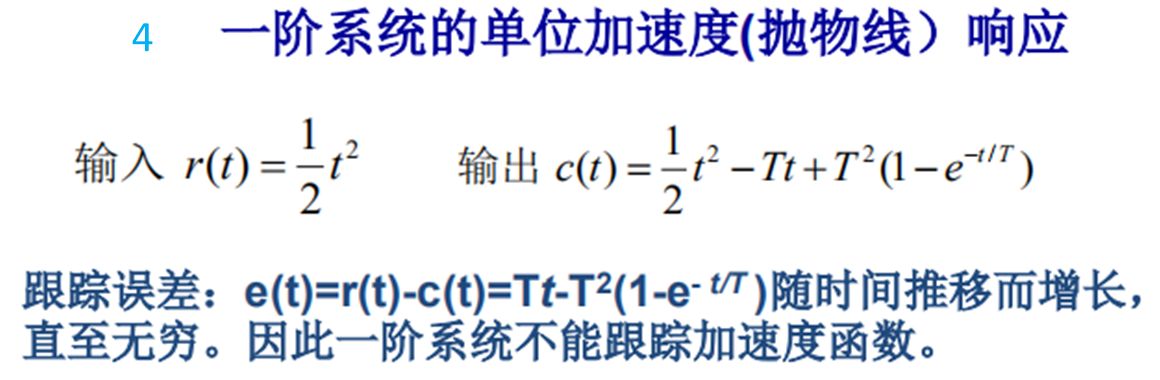3. 时间常数对时间响应的影响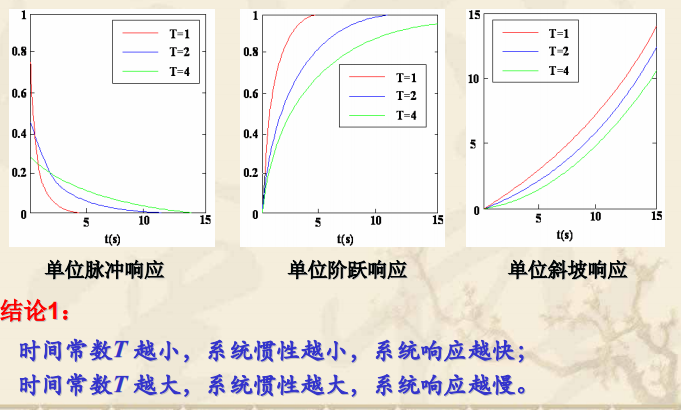4. 一阶系统性能指标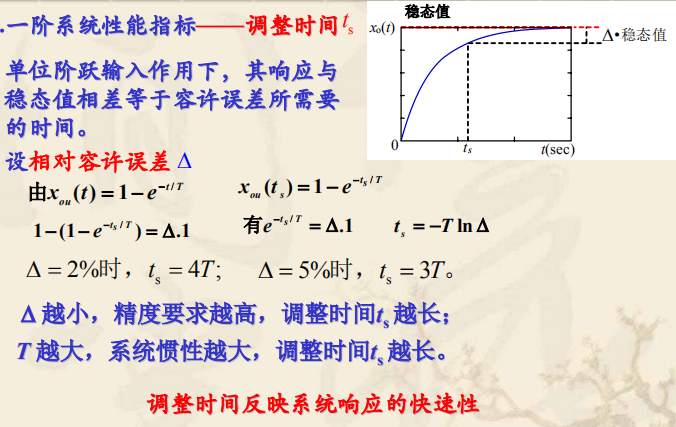展开全文• 一阶系统的时域分析一阶系统的数学模型一阶系统的单位阶跃响应改善系统性能的简单方法 可以用一阶微分方程描述的系统，称为一阶系统。如RC网络、空气加热系统、液面控制系统等都可以用一阶或近似一阶系统来表示。 ...


一阶系统的时域分析
一阶系统的数学模型一阶系统的单位阶跃响应改善系统性能的简单方法通过负反馈减小时间常数在系统的前向通道上串联一个比例环节

可以用一阶微分方程描述的系统，称为一阶系统。如RC网络、空气加热系统、液面控制系统等都可以用一阶或近似一阶系统来表示。
一阶系统的数学模型获得了一阶系统的数学模型后，我们将分别就不同的典型输入信号，求解该系统的时域响应，并据此对系统的性能进行分析。
一阶系统的单位阶跃响应一阶系统的单位阶跃响应是一条由零开始按指数规律单调上升并最终趋于1的曲线，响应曲线具有非振荡特性。
一阶系统的单位阶跃响应在不同时刻的输出值可以列表如下:时间常数T反映了系统的惯性，时间常数T越大，表示系统的惯性越大，响应速度越慢，系统跟踪单位阶跃信号越慢，单位阶跃响应曲线上升越缓。反之，惯性越小，响应速度越快，系统跟踪单位阶跃信号越快，单位阶跃响应曲线.上升越陡峭。改善系统性能的简单方法
通过负反馈减小时间常数对一阶系统而言，反馈加深可使调节时间减小。但随着反馈加深，系统输出的幅值逐渐减小。
在系统的前向通道上串联一个比例环节展开全文性能 时域
• matlab开发-一阶系统调整新理论。一阶系统调整（李亚普诺夫理论）Simulink基础
• labview 做的一阶系统响应，列出了系统原理框图，简要介绍了本系统为自动控制原理中的一阶系统典型环节虚拟实验系统，当输入不同的K值和T值时，可以确定不同的传递函数，据此可以画出一阶系统惯性环节的单位阶跃响应...
• matlab开发-一阶系统适应度的确定。一阶系统（自适应增益的确定）Simulink基础
• matlab开发-一阶系统调整规范化指令。一阶系统调整（标准化MIT规则）Simulink基础
• 通过简单RLC元件构建一阶电路和二阶电路，物理数学仿真进行分析。
• 最小二乘法辨识一阶系统-lsq.m 在控制系统的设计过程中，需要被控对象的数学模型 这有一个简单的辨识一阶系统的程序matlab
问题描述

x

˙

=

f

(

x

,

t

)

+

u

\dot{x} = f(x,t)+u

其中

f

f

表示系统所受的总扰动，包含未知的外扰和未建模的内部动态。
这里的仿真实验中测试的总扰动为：

f

(

x

,

t

)

=

x

2

+

0.5

sign

(

sin

⁡

(

2

t

)

)

+

cos

⁡

(

x

t

)

f(x,t) = x^2 + 0.5 \, \text{sign}(\sin(2t)) + \cos(xt)

而且控制器不应该知道该具体形式。
🤷‍♀️
控制目标为使得

x

x

输出如下形状的信号：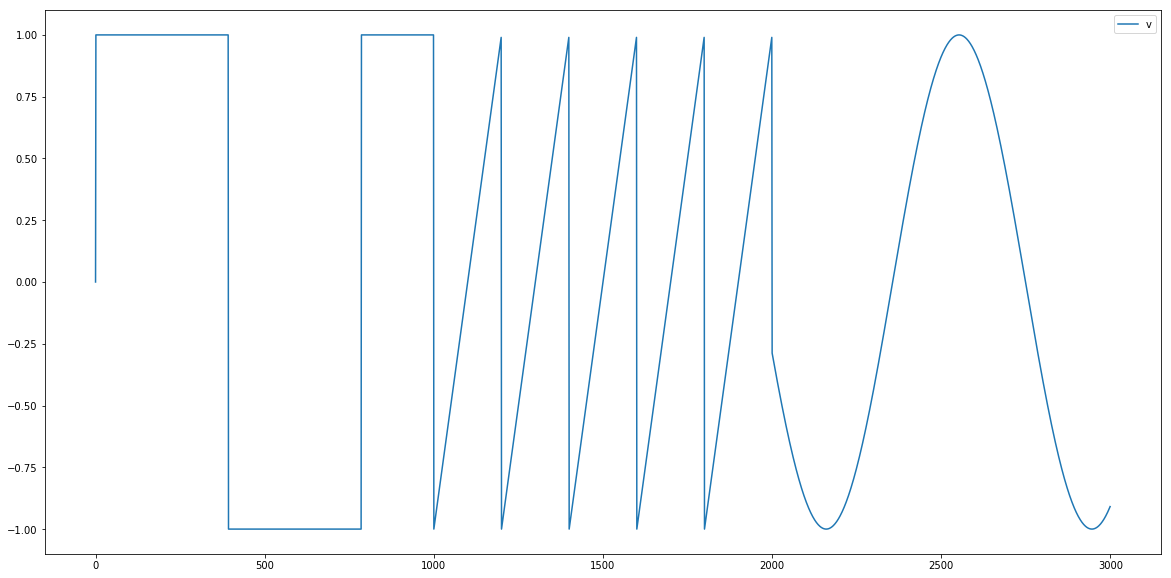代码
总扰动和目标信号
def f(x,t):
return x**2 + 0.5*np.sign(np.sin(2*t)) + np.cos(x*t)

def target(t):
if t < 10:
return np.sign(np.sin(0.8*t))
elif t < 20:
return 2*(0.5*t-int(0.5*t)-0.5)
else:
return np.sin(0.8*t)

定义一个类保存时间序列，方便画图
class Logger:
def __init__(self):
self.data = {}

if key not in self.data:
self.data[key] = []
self.data[key].append(value)

def plot(self, keys=None):
plt.figure(figsize=(20,10))
if keys is None:
for k in self.data:
plt.plot(self.data[k], label=k)
else:
for k in keys:
plt.plot(self.data[k], label=k)
plt.legend()
plt.show()

一个常用的非线性误差函数，具有小误差大增益，大误差小增益的特性。
def fal(x, alpha=0.5, delta=0.1):
return  x/np.power(delta,1-alpha) if np.abs(x)<delta else np.power(np.abs(x), alpha)*np.sign(x)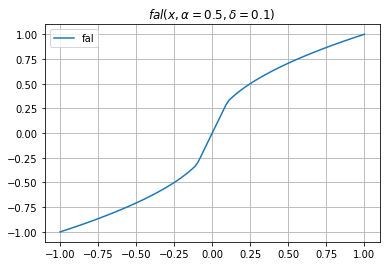使用欧拉积分做数值积分
logger = Logger()
h = 0.01
T = 30
N = int(T/h)

r0 = 5  # r0调控过渡的快慢

# 一些初值的设定
v1 = 0
z1,z2 = 0,0
x = 0
y = x
u = 0
for i in range(N):
t = i*h

# 目标信号
v = target(t)

# 过渡过程 v1 -> v, v1的过渡比v更加平缓
v1 += h*r0*fal(v-v1, 0.5, h)

# ESO: z1 -> y, z2 = \dot{z1}
e = y - z1
fe = fal(e, 0.5, h)
z1 += h*(z2 + 100*e + u)
z2 += h*(300*fe)

# 误差反馈
# e1 = v1 - y
e1 = v1 - z1
u = 10*fal(e1,0.5,0.1) - z2

# 系统状态更新
w = f(x,t)
x += h*(w + u)

# 输出
y = x

logger.plot(['v'])
logger.plot(['v','v1','y'])
logger.plot(['w','z2','u'])

结果展示如下，v代表真实目标信号，

v

1

v_1

是v的平滑过渡，y是实际输出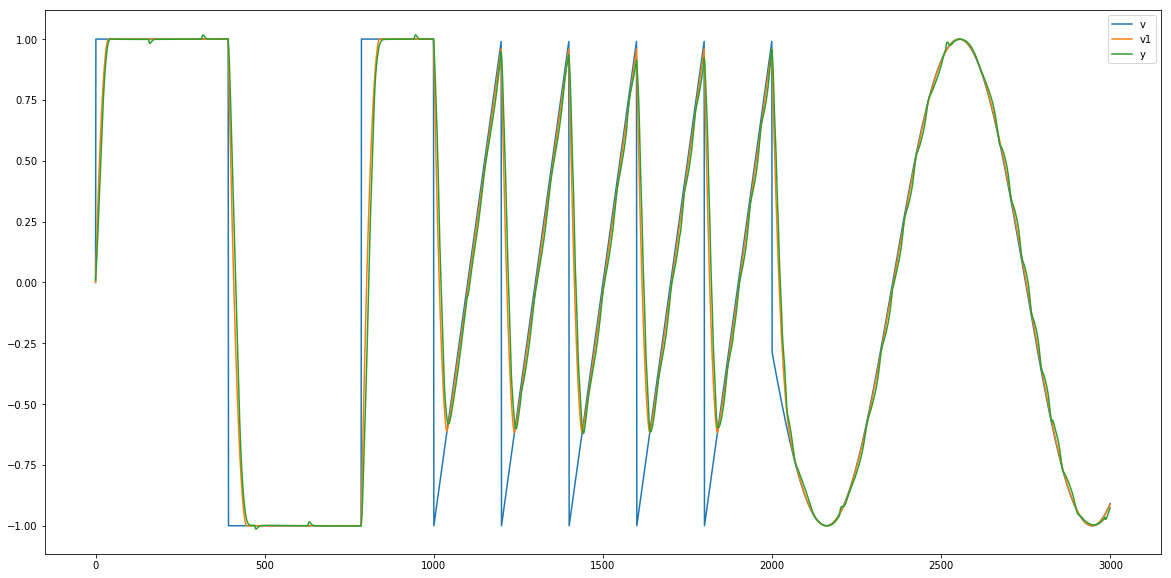w 代表真实总扰动，

z

2

z_2

是对总扰动的跟踪，u是控制输入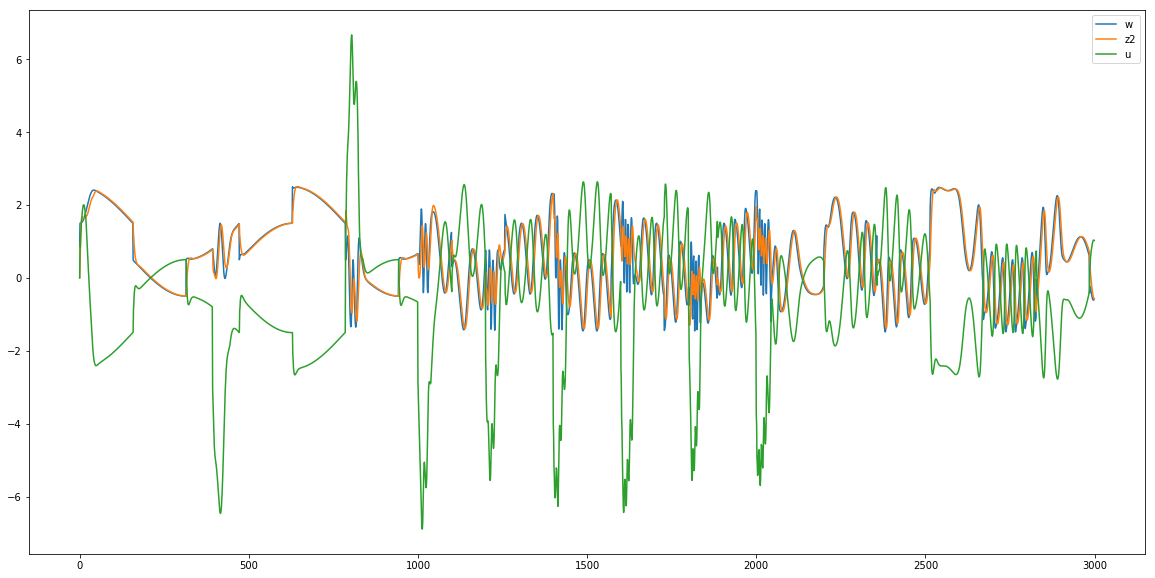下面我来增大

r

0

:

5

→

10

r_0:5\rightarrow 10

，意味着过渡信号

v

1

v_1

更加逼近真实信号

v

v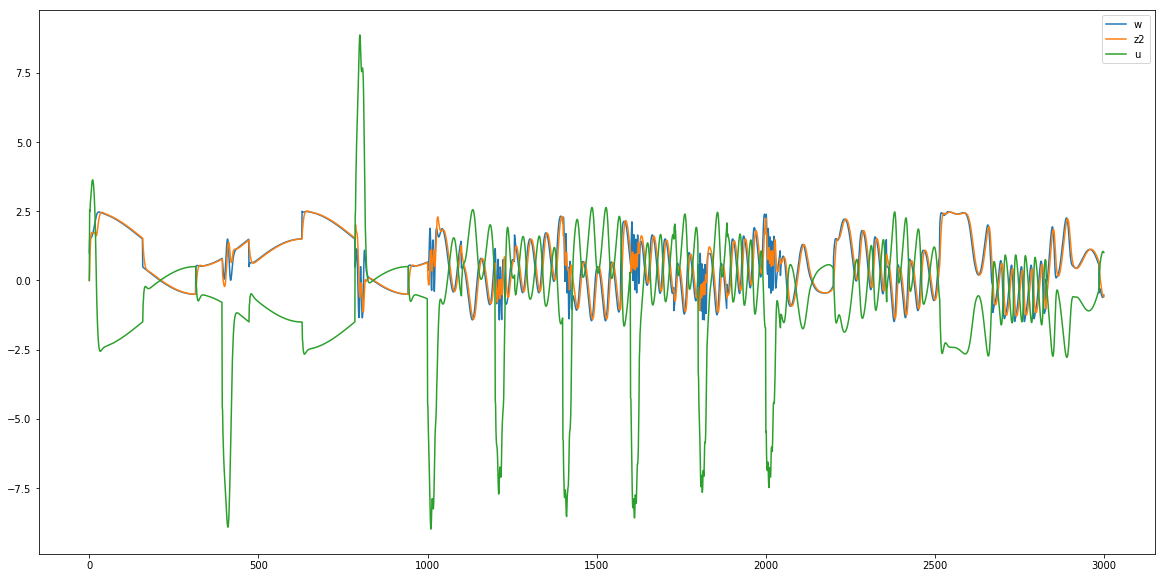过渡过程越短，意味着要在更短的时间做出同样的变化，自然就要求控制量

u

u

的绝对值要增大，从上图可以看出这一点。
展开全文ADRC 自抗扰控制 python
• 1.一阶系统 定义：一阶微分方程描述的系统称为一阶系统 一阶系统： 对该式进行化简-->> 在坐标系中表示为： 该一阶系统的频率特性为： 即对其进行求模运算-->> 对进行分析如下： ...
本次知识学习来源：
微信公众号：maobitcoder

1.一阶系统
定义：一阶微分方程描述的系统称为一阶系统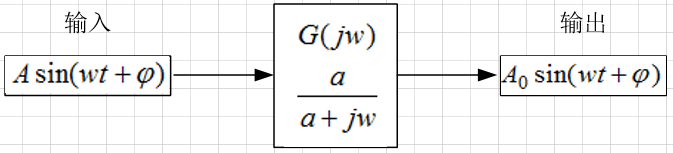一阶系统：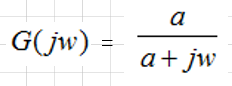对该式进行化简-->>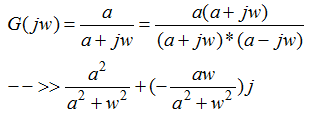在坐标系中表示为：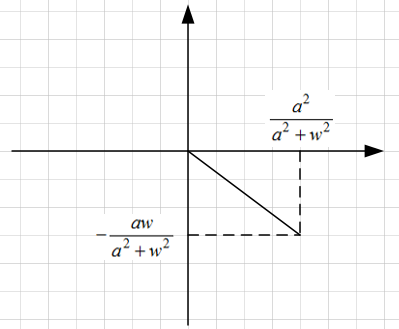该一阶系统的频率特性为：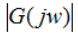即对其进行求模运算-->>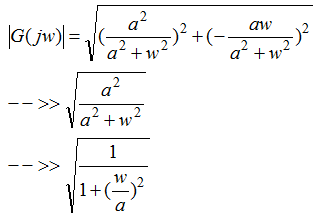对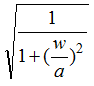进行分析如下：
（1）w很小时，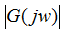约等于1。
（2）w很大时，约等于0。
（3）w等于a时，等于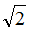（3dB）。
关系图：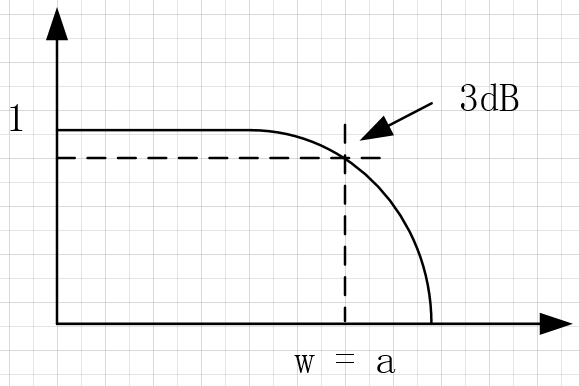2.低通滤波
简易的一阶RC低通滤波器：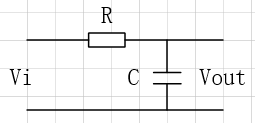截止频率：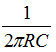关系图：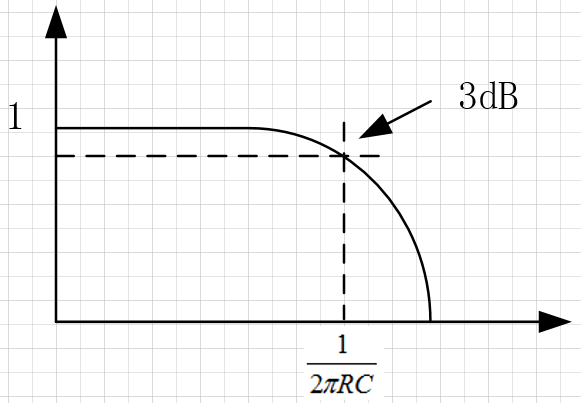总结：此时数学上的一阶系统与电路中的RC低通滤波联系起来。
仿真程序：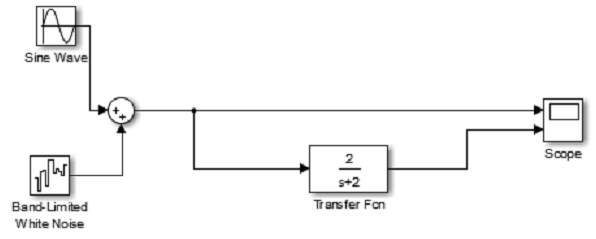仿真结果：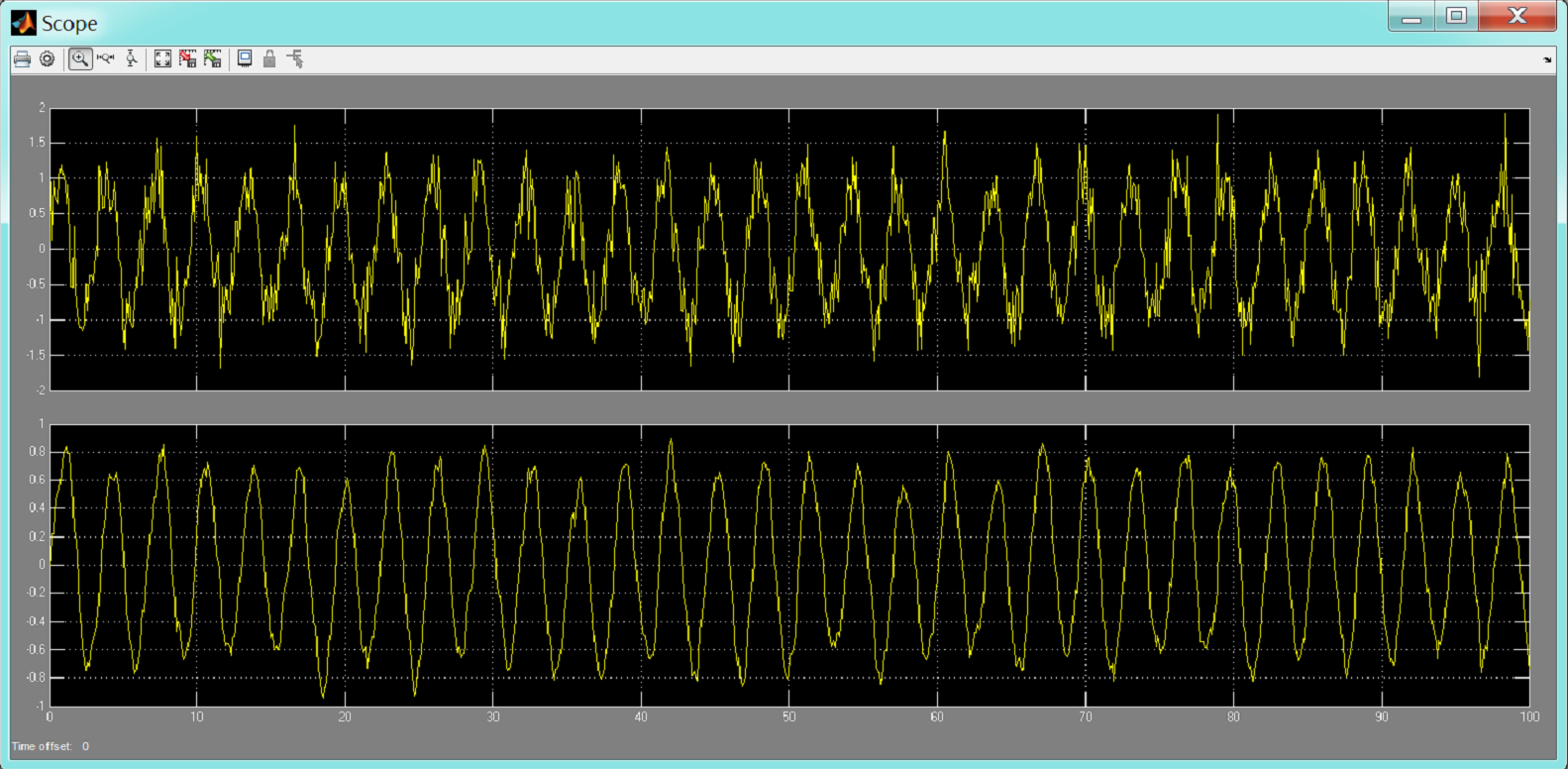展开全文• 基于数值积分的一阶系统时间常数测量方法，田岱琳，周佳，针对传统测量一阶系统特性参数要求系统具有单位灵敏度的缺点，讨论了一种基于数字积分的一阶系统特性参数的估计方法，并给出了数
• 1、一阶系统传递函数的标准形式 一阶系统：系统传递函数的分子分母的最高次数都不超过1次。 一阶系统的典型结构如图3-2所示，K是开环增益。 其开环传函：G(s)=KsG_{(s)}=\frac{K}{s}G(s)​=sK​ 闭环传函：Φ(s)=G...
•研究论文
• 先来简述一阶系统(也可直接跳转至2部分)积分器+单位负反馈组成的一阶系统框图闭环传递函数框图的伯德图阶跃响应阶跃响应(时域响应)带宽处幅值下降3dB≈√2增益-带宽积一节运放的传递函数单位增益频率(unit-gain ...
• 上图的波形是对一个电感充电5us，放电后产生的阻尼振荡波形。 具体电路： 一个充满电的电容或电感、然后一个二阶系统RLC，测量电容或电感上的电压就实现了。 二阶系统和一阶系统
• 一阶系统的时域分析 前言 在经典控制理论中，常用时域分析法、根轨迹法或频域分析法来分析线性控制系统的性能，不同的方法有不同的特点和适用范围，但是比较而言，时域分析法是一种直接在时间域中对系统进行分析的...自动控制原理
• [分布式控制浅述] （2） 经典一阶系统的一致性问题[分布式控制浅述] （2） 经典一阶系统的一致性问题1 前言2 经典一阶系统的一致性问题2 关于均值的推论 [分布式控制浅述] （2） 经典一阶系统的一致性问题 对于多...
• 一阶系统的matlab分析 上图是用simulink仿真的一阶系统对阶跃型号的响应 传递函数可以简化为theta(s) = K/(Ts+1) 为方便研究，K为常数，令K等于1。 探究不同的T情况下，一阶系统对阶跃激励的响应。 代码很...matlab
• 系列文章目录 自动控制理论（1）——自动控制理论概述 ...稳态性能指标二、一阶系统时域分析1.数学模型2.单位阶跃响应3.单位斜坡响应4.单位脉冲响应5.总结 前言 时域分析：根据控制系统的时间响应来分析系统的稳定
• 关于一阶系统的PID 算法控制的仿真设计一、设计内容对一阶系统实现PID 算法控制并进行仿真，具体功能如下：基本要求：实现PID 算法和一阶系统差分方程仿真，PID 算法中的四个参数和一阶系统的参数都可以通过菜单进行...
• % 一阶惯性滞后系统辨识 - 切线辨识 dt = 0.01; tmax = 20; t=0:dt:tmax; s = tf('s'); % 设定待辨识传递函数 k0=6; T =3; tau=2; H=k0/(T*s+1); %参数 τ=2,K=6,T=3 H.InputDelay=tau; %待辨识...
• 金鸡一唱天下白：第三讲 控制系统的复域数学模型(传递函数)​... 由具有一定函数关系的环节组成的，并标明信号流向的系统的方框图，称为系统的结构图。系统的结构图实质上是系统原理图与传递函数两者的综合。可以......